## Involuntary

A Linear Transformation of period two. Since a Linear Transformation has the form,(1)

applying the transformation a second time gives(2)

For an involuntary,, so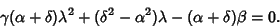(3)

Since each Coefficient must vanish separately,(4)(5)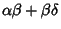(6)

The first equation gives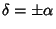. Taking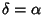would require, giving, the identity transformation. Takinggives, so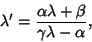(7)

the general form of an Involution.